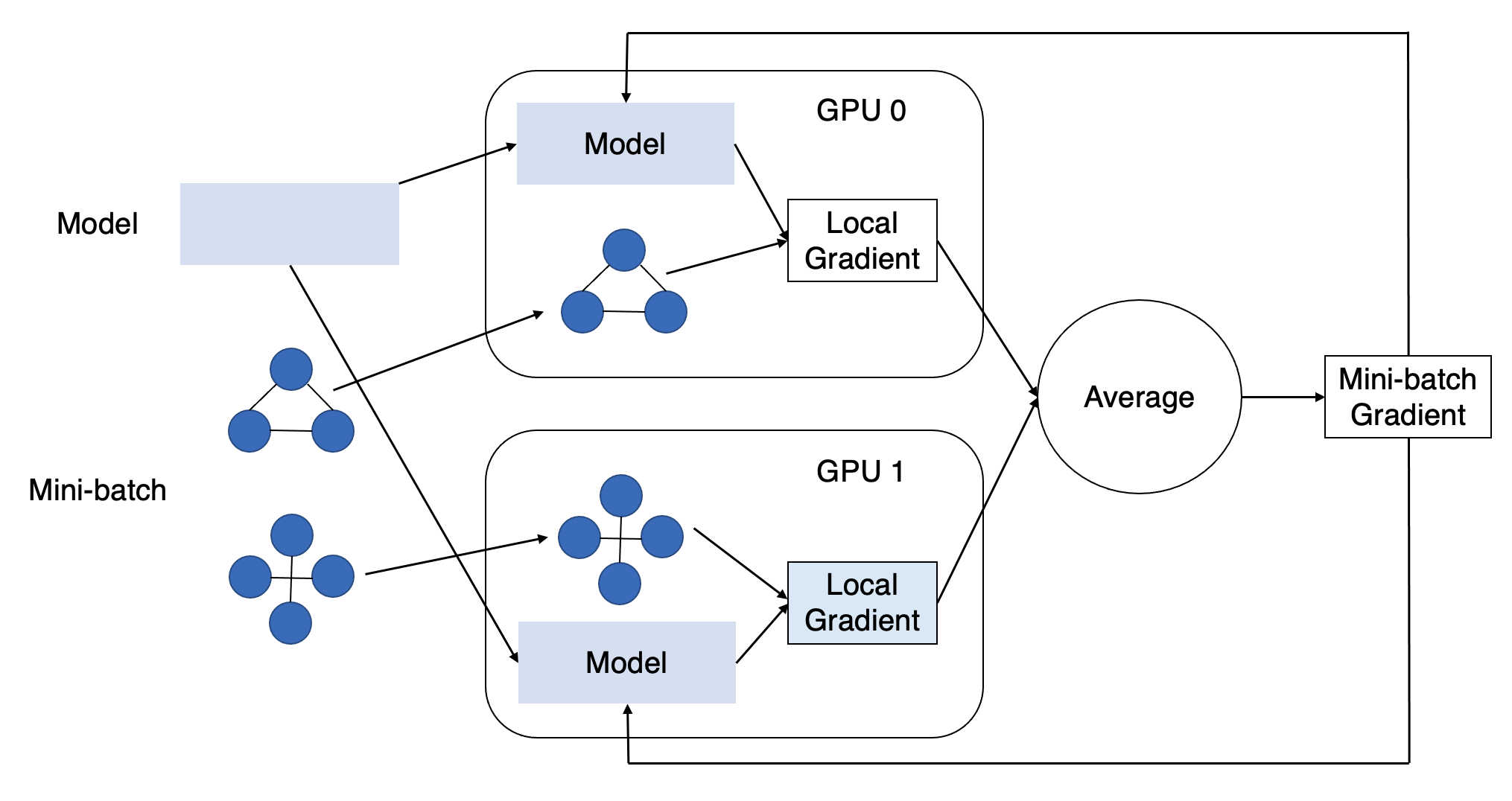# Single Machine Multi-GPU Minibatch Graph Classification¶

In this tutorial, you will learn how to use multiple GPUs in training a graph neural network (GNN) for graph classification. This tutorial assumes knowledge in GNNs for graph classification and we recommend you to check Training a GNN for Graph Classification otherwise.

(Time estimate: 8 minutes)

To use a single GPU in training a GNN, we need to put the model, graph(s), and other tensors (e.g. labels) on the same GPU:

import torch

# Use the first GPU
device = torch.device("cuda:0")
model = model.to(device)
graph = graph.to(device)
labels = labels.to(device)


The node and edge features in the graphs, if any, will also be on the GPU. After that, the forward computation, backward computation and parameter update will take place on the GPU. For graph classification, this repeats for each minibatch gradient descent.

Using multiple GPUs allows performing more computation per unit of time. It is like having a team work together, where each GPU is a team member. We need to distribute the computation workload across GPUs and let them synchronize the efforts regularly. PyTorch provides convenient APIs for this task with multiple processes, one per GPU, and we can use them in conjunction with DGL.

Intuitively, we can distribute the workload along the dimension of data. This allows multiple GPUs to perform the forward and backward computation of multiple gradient descents in parallel. To distribute a dataset across multiple GPUs, we need to partition it into multiple mutually exclusive subsets of a similar size, one per GPU. We need to repeat the random partition every epoch to guarantee randomness. We can use GraphDataLoader(), which wraps some PyTorch APIs and does the job for graph classification in data loading.

Once all GPUs have finished the backward computation for its minibatch, we need to synchronize the model parameter update across them. Specifically, this involves collecting gradients from all GPUs, averaging them and updating the model parameters on each GPU. We can wrap a PyTorch model with DistributedDataParallel() so that the model parameter update will invoke gradient synchronization first under the hood.That’s the core behind this tutorial. We will explore it more in detail with a complete example below.

Note

See this tutorial from PyTorch for general multi-GPU training with DistributedDataParallel.

## Distributed Process Group Initialization¶

For communication between multiple processes in multi-gpu training, we need to start the distributed backend at the beginning of each process. We use world_size to refer to the number of processes and rank to refer to the process ID, which should be an integer from 0 to world_size - 1.

import torch.distributed as dist

def init_process_group(world_size, rank):
dist.init_process_group(
backend='gloo',     # change to 'nccl' for multiple GPUs
init_method='tcp://127.0.0.1:12345',
world_size=world_size,
rank=rank)


We split the dataset into training, validation and test subsets. In dataset splitting, we need to use a same random seed across processes to ensure a same split. We follow the common practice to train with multiple GPUs and evaluate with a single GPU, thus only set use_ddp to True in the GraphDataLoader() for the training set, where ddp stands for DistributedDataParallel().

from dgl.data import split_dataset

# Use a 80:10:10 train-val-test split
train_set, val_set, test_set = split_dataset(dataset,
frac_list=[0.8, 0.1, 0.1],
shuffle=True,
random_state=seed)



## Model Initialization¶

For this tutorial, we use a simplified Graph Isomorphism Network (GIN).

import torch.nn as nn
import torch.nn.functional as F
from dgl.nn.pytorch import GINConv, SumPooling

class GIN(nn.Module):
def __init__(self, input_size=1, num_classes=2):
super(GIN, self).__init__()

self.conv1 = GINConv(nn.Linear(input_size, num_classes), aggregator_type='sum')
self.conv2 = GINConv(nn.Linear(num_classes, num_classes), aggregator_type='sum')
self.pool = SumPooling()

def forward(self, g, feats):
feats = self.conv1(g, feats)
feats = F.relu(feats)
feats = self.conv2(g, feats)

return self.pool(g, feats)


To ensure same initial model parameters across processes, we need to set the same random seed before model initialization. Once we construct a model instance, we wrap it with DistributedDataParallel().

import torch
from torch.nn.parallel import DistributedDataParallel

def init_model(seed, device):
torch.manual_seed(seed)
model = GIN().to(device)
if device.type == 'cpu':
model = DistributedDataParallel(model)
else:
model = DistributedDataParallel(model, device_ids=[device], output_device=device)

return model


## Main Function for Each Process¶

Define the model evaluation function as in the single-GPU setting.

def evaluate(model, dataloader, device):
model.eval()

total = 0
total_correct = 0

bg = bg.to(device)
labels = labels.to(device)
# Get input node features
feats = bg.ndata.pop('attr')
pred = model(bg, feats)
_, pred = torch.max(pred, 1)
total += len(labels)
total_correct += (pred == labels).sum().cpu().item()

return 1.0 * total_correct / total


Define the main function for each process.

from torch.optim import Adam

def main(rank, world_size, dataset, seed=0):
init_process_group(world_size, rank)
if torch.cuda.is_available():
device = torch.device('cuda:{:d}'.format(rank))
torch.cuda.set_device(device)
else:
device = torch.device('cpu')

model = init_model(seed, device)
criterion = nn.CrossEntropyLoss()

seed)
for epoch in range(5):
model.train()
# The line below ensures all processes use a different

total_loss = 0
bg = bg.to(device)
labels = labels.to(device)
feats = bg.ndata.pop('attr')
pred = model(bg, feats)

loss = criterion(pred, labels)
total_loss += loss.cpu().item()
loss.backward()
optimizer.step()
loss = total_loss
print('Loss: {:.4f}'.format(loss))

print('Val acc: {:.4f}'.format(val_acc))

print('Test acc: {:.4f}'.format(test_acc))
dist.destroy_process_group()


Finally we load the dataset and launch the processes.

if __name__ == '__main__':
import torch.multiprocessing as mp

from dgl.data import GINDataset

num_gpus = 4
procs = []
dataset = GINDataset(name='IMDBBINARY', self_loop=False)
mp.spawn(main, args=(num_gpus, dataset), nprocs=num_gpus)

# Thumbnail credits: DGL
# sphinx_gallery_thumbnail_path = '_static/blitz_5_graph_classification.png'


Total running time of the script: ( 0 minutes 0.004 seconds)

Gallery generated by Sphinx-Gallery